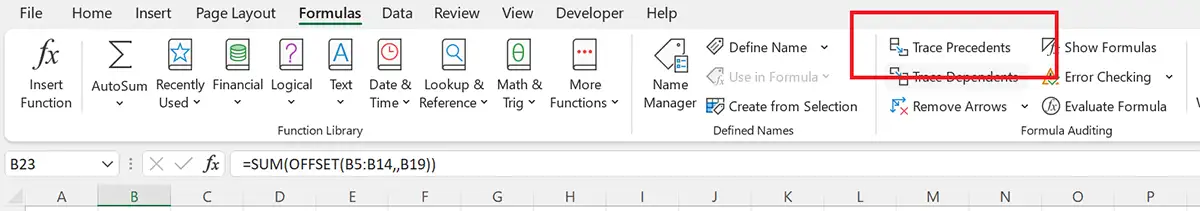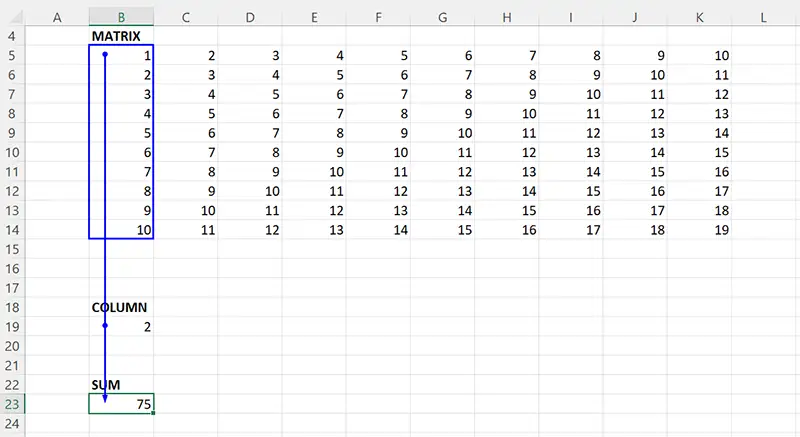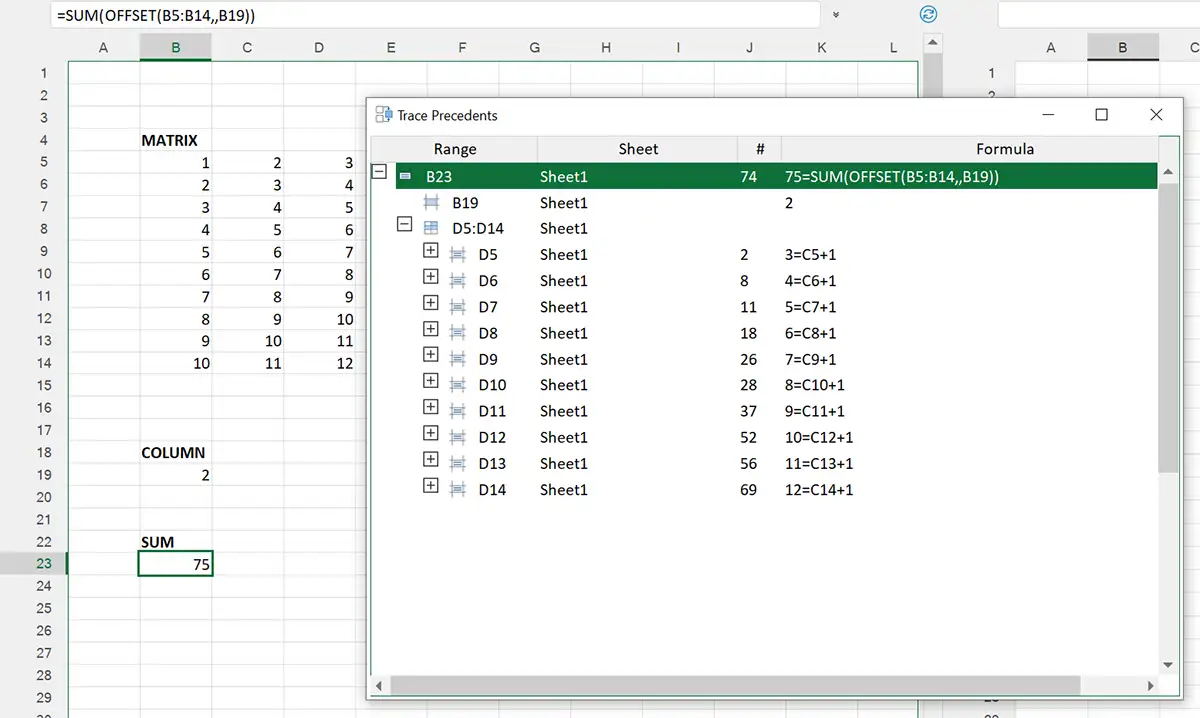﻿ Trace Precedents in Excel

# How to Trace Precedents in Excel file

Complex Excel files contains thousands of formulas. We would like to talk, how to effectively work with large number of calculations.## What is Excel formula?

Formula is an expression that operates ranges of cells as arguments
Excel allows you to use number of functions, like SUM, PRODUCT, OFFSET, etc., arithmetic and comparison operations. Also, you can write your own formula function using VBA language.

## What is precedent in Excel formula?

Precedent is a cell (range) that is used as argument in a formula.
Example. In cell G12 you have formula:
=SUM(K12:Z12)
Cells in range K12:Z12 are precedents of the cell G12.
G12 is called dependent for range K12:Z12.

## How to see all precedents of my formula on Excel worksheet?

Standard method:
2. Go to Formulas tab on the Ribbon
3. Use Trace Dependents commandExcel points you to precedents with blue arrows that go from arguments to selected cell.This is perfect visual representation of the dependency between cells, involved into specific formula.

1. Open your file in the xlCompare
2. Select cell, whose precedents you are interested in.
3. Use Tools | Trace Dependents command on the Ribbon.
4. xlCompare displays you list of precedents in a form of list.## What are the benefits of using xlCompare to Trace Precedents?

1. You are getting all ranges as single list
2. You see defined names in this list
3. You can expand every cell in this list and Trace Precedents recursively
4. You can drill down into precedents until you get input cells in your model
5. Choose any precedent range in list and xlCompare will select it on worksheet
Is this method better than in Excel? This is just an alternative. If it is more suitable for you - use xlCompare. Otherwise you can highlight precedents in Excel.

## Trace Indirect Precedents in Excel file

Imagine that you have following formula:
=SUM(OFFSET(B5:B14,,B19))
Excel shows you B5:B14 as precedents, but these cells are not used in the calculations! This is just a starting point of the OFFSET function.
xlCompare gives you D5:D14! This is indirect precedents. This is dependency created dynamically when your formula is calculated. It is now known before.
xlCompare shows you actual precedents in your model!
This feature is available for free. You should not pay for it.
So, can be very handy addition to your copy of the Microsoft Excel 365.
xlCompare is more than Excel Diff Tool!